Data


library(PointFore)
#>
#> Attaching package: 'PointFore'
#> The following object is masked from 'package:stats':
#>
#>     lag
library(ggplot2)

ggplot(GDP)+
geom_line(aes(x=date,y=observation))+
geom_point(aes(x=date,y=forecast), color = 'red', size = 2, shape=4)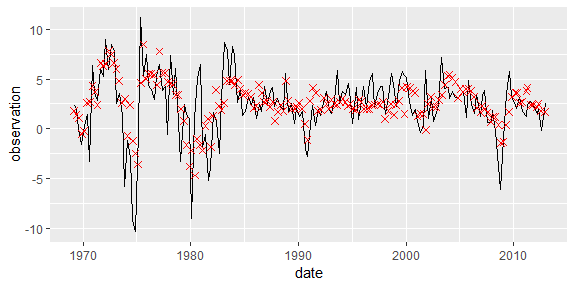Time series of one-quarter ahead Greenbook forecasts (crosses) and respective observations (solid line) of real GDP growth in the US.

Next to this forecast, which was generated by taking the forecast issued closest to the midpoint of the quarter before the considered quarter, we also consider the forecast issued latest in the respective quarter.


ggplot(GDP)+
geom_line(aes(x=date,y=forecast-forecast_late), color = 'red',alpha=.5)+
geom_point(aes(x=date,y=forecast-forecast_late), color = 'red', size = .5, shape=4)+
ylim(-10,10)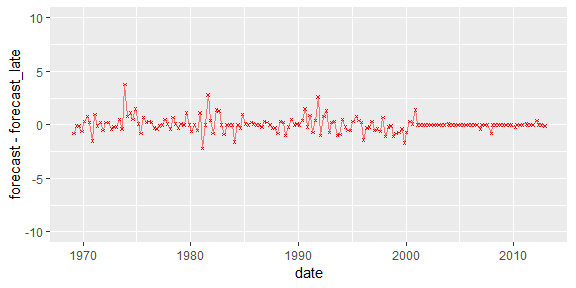Time series of difference between the two considered forecasts.

We see there is considerable adjustement. Starting 1980, there are normally 2 instead of 3 forecasts issued each quarter. The publication dates vary across time.

Analysis

Now, let us analyse the forecasts. First, we consider the forecast issued closest to the midpoint of the respective quarter. Then, we analyze the latest issued forecast with potentially more information.

We begin by estimating and testing constant quantile forecasts. First, for the main forecast:


res <- estimate.functional(model = constant,
state = GDP$forecast, Y=GDP$observation,X=GDP$forecast) #> Drop 1 case(s) because of chosen instruments #> Choose parameter theta0 automatically. summary(res) #>$call
#> estimate.functional(model = constant, Y = GDP$observation, X = GDP$forecast,
#>     stateVariable = GDP$forecast) #> #>$coefficients
#>           Estimate Std. Error  t value     Pr(>|t|)
#> Theta 0.5980637 0.04429534 13.50173 1.527435e-41
#>
#> $Jtest #> #> ## J-Test: degrees of freedom is 2 ## #> #> J-test P-value #> Test E(g)=0: 5.507506 0.063688 Optimality is rejected with a p-value of 0.06. If we consider less restrictive information sets, the same holds true.  res <- estimate.functional(model = constant, instruments = c("lag(lag(Y))","X"), state = GDP$forecast, Y=GDP$observation,X=GDP$forecast)
#> Drop  2 case(s) because of chosen instruments
#> Choose parameter theta0 automatically.
summary(res)
#> $call #> estimate.functional(model = constant, Y = GDP$observation, X = GDP$forecast, #> stateVariable = GDP$forecast, instruments = c("lag(lag(Y))",
#>         "X"))
#>
#> $coefficients #> Estimate Std. Error t value Pr(>|t|) #> Theta 0.6013492 0.04349651 13.82523 1.795448e-43 #> #>$Jtest
#>
#>  ##  J-Test: degrees of freedom is 2  ##
#>
#>                 J-test    P-value
#> Test E(g)=0:    6.098404  0.047397

However, if we consider the forecast issued later in the quarter, the tests cannot reject optimality.


res <- estimate.functional(model = constant,
state = GDP$forecast_late, Y=GDP$observation,X=GDP$forecast_late) #> Drop 1 case(s) because of chosen instruments #> Choose parameter theta0 automatically. summary(res) #>$call
#> estimate.functional(model = constant, Y = GDP$observation, X = GDP$forecast_late,
#>     stateVariable = GDP$forecast_late) #> #>$coefficients
#>           Estimate Std. Error  t value     Pr(>|t|)
#> Theta 0.5842538 0.04086895 14.29579 2.324649e-46
#>
#> $Jtest #> #> ## J-Test: degrees of freedom is 2 ## #> #> J-test P-value #> Test E(g)=0: 2.47565 0.29001 car::linearHypothesis(res$gmm,"Theta=.5")
#> Linear hypothesis test
#>
#> Hypothesis:
#> Theta = 0.5
#>
#> Model 1: restricted model
#> Model 2: res$gmm #> #> Df Chisq Pr(>Chisq) #> 1 #> 2 1 4.25 0.03925 * #> --- #> Signif. codes: 0 '***' 0.001 '**' 0.01 '*' 0.05 '.' 0.1 ' ' 1 Noteworthy, the median forecast is still rejected, which is indicative of an optimal but asymmetric forecast. In the following we try to understand under which conditions the main forecast may be optimal. Replication of the results in “Interpretation of Point Forecasts with Unknown Directive” by Schmidt, Katzfuss and Gneiting First, we replicate the main result from the original paper.  res <- estimate.functional(model = probit_linear, state = GDP$forecast, Y=GDP$observation,X=GDP$forecast)
#> Drop  1 case(s) because of chosen instruments
#> Choose parameter theta0 automatically.
summary(res)
#> $call #> estimate.functional(model = probit_linear, Y = GDP$observation,
#>     X = GDP$forecast, stateVariable = GDP$forecast)
#>
#> $coefficients #> Estimate Std. Error t value Pr(>|t|) #> Theta -0.1125011 0.16807744 -0.6693408 0.50327812 #> Theta 0.1132529 0.04437854 2.5519745 0.01071144 #> #>$Jtest
#>
#>  ##  J-Test: degrees of freedom is 1  ##
#>
#>                 J-test   P-value
#> Test E(g)=0:    1.38747  0.23883
plot(res,hline = TRUE)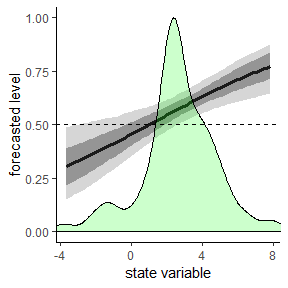State-dependent quantile analysis with a linear model (with probit) link that depends on the current forecast.

The evidence can be summarized as follows. The forecast indicates overshooting in the presence of extreme expected growth. There is strong evidence of overshooting in times of high growth (current forecast > 3) and weak evidence in times of low growth (current forecast < 0, recession expected). Further, there is no evidence that the forecast is sub-optimal as the optimality test has a p-value of 0.24. Hence, the assumption that the forecast is generate as state-dependent quantile $X_t = q_{m(X_{t},\theta)}(Y_t|\mathcal{F}_t).$ seems valid.

Further, there is evidence that the forecasted level actually depends on the current forecast as the Wald-test for $$\theta_2=0$$ has a p-value of 0.011. That is the assumption that the forecast is generate as optimal constant quantile forecast does not seem to hold:

$X_t = q_{\tau}(Y_t|\mathcal{F}_t).$

Different information sets (instrumental vectors)

In the following we apply the same analysis with different instrumental vectors. The standard in the estimate.functional function is c(“lag(Y)”,“X”) which signifies that the instruments are given by $w_t=(1,y_{t-1},x_t)'.$

As first alternative, we consider only lagged values as instruments. That is the instruments are given by $w_t=(1,y_{t-1},y_{t-2})'.$


res <- estimate.functional(model = probit_linear, instruments = c("lag(Y)","lag(lag(Y))"),
state = GDP$forecast, Y=GDP$observation,X=GDP$forecast) #> Drop 2 case(s) because of chosen instruments #> Choose parameter theta0 automatically. summary(res) #>$call
#> estimate.functional(model = probit_linear, Y = GDP$observation, #> X = GDP$forecast, stateVariable = GDP$forecast, instruments = c("lag(Y)", #> "lag(lag(Y))")) #> #>$coefficients
#>            Estimate Std. Error   t value  Pr(>|t|)
#> Theta 0.05953213 0.20481276 0.2906661 0.7713067
#> Theta 0.04941455 0.06520126 0.7578771 0.4485245
#>
#> $Jtest #> #> ## J-Test: degrees of freedom is 1 ## #> #> J-test P-value #> Test E(g)=0: 0.00015956 0.98992153 plot(res,hline = TRUE)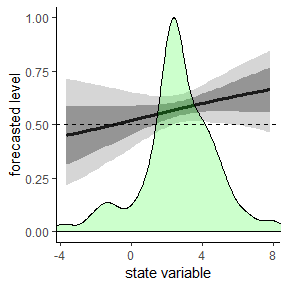State-dependent quantile analysis with a linear model (with probit link) that depends on the current forecast with instruments $$w_t=(1, Y_{t-1}, Y_{t-2})$$. As argued in the main paper, this choice often lags power against identifying misspecified models. And indeed, we observe large confidence bands and a high p-value indicating that the model fits well. Arguably this is because the instruments generate a rather limited information set, such that forecast optimality with respect to this limited information set is a weak requirement. As second alternative, we consider that the lagged outcome $$Y_{t-1}$$ may not be in the information set of the forecast. As instruments we use just the forecasts $W_t = \left( 1, X_t, X_{t-1} \right)' '.$  res <- estimate.functional(model = probit_linear, instruments = c( "X", "lag(X)"), state = GDP$forecast, Y=GDP$observation,X=GDP$forecast)
#> Drop  1 case(s) because of chosen instruments
#> Choose parameter theta0 automatically.
summary(res)
#> $call #> estimate.functional(model = probit_linear, Y = GDP$observation,
#>     X = GDP$forecast, stateVariable = GDP$forecast, instruments = c("X",
#>         "lag(X)"))
#>
#> $coefficients #> Estimate Std. Error t value Pr(>|t|) #> Theta -0.1161105 0.16685478 -0.6958775 0.48650555 #> Theta 0.1142752 0.04518285 2.5291727 0.01143317 #> #>$Jtest
#>
#>  ##  J-Test: degrees of freedom is 1  ##
#>
#>                 J-test   P-value
#> Test E(g)=0:    0.23589  0.62719
plot(res,hline = TRUE)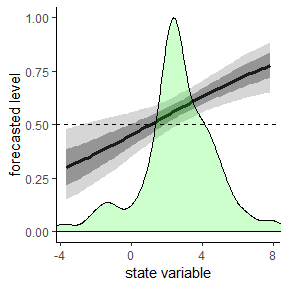State-dependent quantile analysis with a linear model (with probit link) that depends on the current forecast with instruments $$w_t=(1, X_t, X_{t-1})$$.

We observe similar results to the main specification in the paper: The test of overidentifying restrictions does not reject optimality, strong evidence of overshooting in times of high expected growth and weak evidence of undershootin in times of low expected growth.

As third alternative, we consider the instrument $W_t = \left( 1, X_t, Y_{t-2} \right).$


res <- estimate.functional(model = probit_linear,
instruments = c( "X", "lag(lag(Y))"),
state = GDP$forecast, Y=GDP$observation,X=GDP$forecast) #> Drop 2 case(s) because of chosen instruments #> Choose parameter theta0 automatically. summary(res) #>$call
#> estimate.functional(model = probit_linear, Y = GDP$observation, #> X = GDP$forecast, stateVariable = GDP$forecast, instruments = c("X", #> "lag(lag(Y))")) #> #>$coefficients
#>            Estimate Std. Error    t value  Pr(>|t|)
#> Theta -0.1042893 0.16823370 -0.6199075 0.5353187
#> Theta  0.1146164 0.04533643  2.5281297 0.0114672
#>
#> $Jtest #> #> ## J-Test: degrees of freedom is 1 ## #> #> J-test P-value #> Test E(g)=0: 0.47321 0.49152 plot(res,hline = TRUE)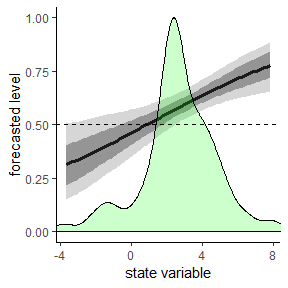State-dependent quantile analysis with a linear model (with probit link) that depends on the current forecast with instruments $$w_t=(1, X_t, Y_{t-2})$$. We observe similar results to the main specification in the paper: The test of overidentifying restrictions does not reject optimality, strong evidence of overshooting in times of high expected growth and weak evidence of undershootin in times of low expected growth. Finally, we consider the larger set of instruments used in the other simulation study in the main paper. That is the instruments are given by $W_t = \left( 1, X_t, X_{t-1} - Y_{t-1}, (X_{t-1} - Y_{t-1})^2, X_{t-1}, X_{t-2} - Y_{t-2}, (X_{t-2} - Y_{t-2})^2 \right)' '.$  res <- estimate.functional(model = probit_linear, instruments = c( "X", "lag(X-Y)", "lag(X-Y)^2", "lag(X)", "lag(lag(X-Y))", "lag(lag(X-Y))^2"), state = GDP$forecast, Y=GDP$observation,X=GDP$forecast)
#> Drop  2 case(s) because of chosen instruments
#> Choose parameter theta0 automatically.
summary(res)
#> $call #> estimate.functional(model = probit_linear, Y = GDP$observation,
#>     X = GDP$forecast, stateVariable = GDP$forecast, instruments = c("X",
#>         "lag(X-Y)", "lag(X-Y)^2", "lag(X)", "lag(lag(X-Y))",
#>         "lag(lag(X-Y))^2"))
#>
#> $coefficients #> Estimate Std. Error t value Pr(>|t|) #> Theta -0.1497501 0.16831514 -0.8897005 0.373626751 #> Theta 0.1202451 0.04513079 2.6643704 0.007713258 #> #>$Jtest
#>
#>  ##  J-Test: degrees of freedom is 5  ##
#>
#>                 J-test   P-value
#> Test E(g)=0:    3.90743  0.56282
plot(res,hline = TRUE)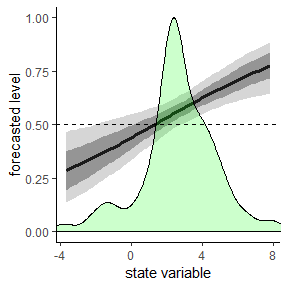State-dependent quantile analysis with a linear model (with probit link) that depends on the current forecast with instruments $$w_t=(1, X_t, X_{t-1} - Y_{t-1}, (X_{t-1} - Y_{t-1})^2, X_{t-1}, X_{t-2} - Y_{t-2}, (X_{t-2} - Y_{t-2})^2$$.

Again, the results are robust.

Different specification models

In this section, we want to allow for other specification models as the linear model with probit link.

First, we consider splines in the state variable. We limit our analysis to quadratic and cubic splines. As the spline models have more parameters, we use again the high dimensional instrumental vector from the simulation setting.


instruments = c( "X", "lag(X-Y)", "lag(X-Y)^2",
"lag(X)", "lag(lag(X-Y))", "lag(lag(X-Y))^2"),
state = GDP$forecast, Y=GDP$observation,X=GDP$forecast) #> Drop 2 case(s) because of chosen instruments #> Choose parameter theta0 automatically. summary(res_quadratic) #>$call
#> estimate.functional(model = probit_spline2, Y = GDP$observation, #> X = GDP$forecast, stateVariable = GDP$forecast, instruments = c("X", #> "lag(X-Y)", "lag(X-Y)^2", "lag(X)", "lag(lag(X-Y))", #> "lag(lag(X-Y))^2")) #> #>$coefficients
#>             Estimate Std. Error    t value  Pr(>|t|)
#> Theta -0.24810759 0.26657220 -0.9307332 0.3519916
#> Theta  0.29123717 0.19491130  1.4942036 0.1351224
#> Theta -0.03327321 0.03127308 -1.0639568 0.2873483
#>
#> $Jtest #> #> ## J-Test: degrees of freedom is 4 ## #> #> J-test P-value #> Test E(g)=0: 2.29437 0.68179 res_cubic <- estimate.functional(model = probit_spline3, instruments = c( "X", "lag(X-Y)", "lag(X-Y)^2", "lag(X)", "lag(lag(X-Y))", "lag(lag(X-Y))^2"), state = GDP$forecast, Y=GDP$observation,X=GDP$forecast)
#> Drop  2 case(s) because of chosen instruments
#> Choose parameter theta0 automatically.
summary(res_cubic)
#> $call #> estimate.functional(model = probit_spline3, Y = GDP$observation,
#>     X = GDP$forecast, stateVariable = GDP$forecast, instruments = c("X",
#>         "lag(X-Y)", "lag(X-Y)^2", "lag(X)", "lag(lag(X-Y))",
#>         "lag(lag(X-Y))^2"))
#>
#> $coefficients #> Estimate Std. Error t value Pr(>|t|) #> Theta -0.475672847 1.03012376 -0.4617628 0.6442514 #> Theta 0.260263938 0.32123756 0.8101915 0.4178301 #> Theta 0.040696203 0.14448208 0.2816696 0.7781969 #> Theta -0.009799494 0.02821846 -0.3472725 0.7283866 #> #>$Jtest
#>
#>  ##  J-Test: degrees of freedom is 3  ##
#>
#>                 J-test   P-value
#> Test E(g)=0:    1.75638  0.62447

plot(res_cubic,hline = TRUE)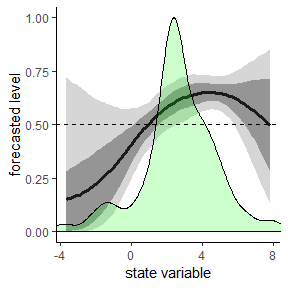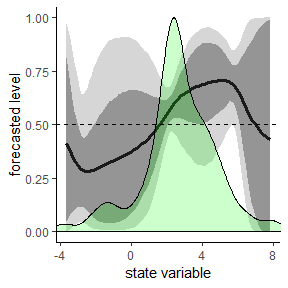The specification models include the linear model as a special case. Hence, it is without surprise that the optimality tests are passed in this case, too. Again we observe that the results are largely in line with the main analysis in the paper in that there is strong evidence for overshooting in times of high expected growth and weaker evidence in times of low growth (or recession). The large confidence bands for more extreme forecasts indicate that the linear model arguably is overly confident for those areas for its strong parametric assumption.

Different state variables

In this section, we use another typical state variable, namely the lagged value of the observation $$Y_{t-1}$$.


res <- estimate.functional(model = probit_linear,
state = lag(GDP$observation), Y=GDP$observation,X=GDP$forecast) #> Drop 1 case(s) because of chosen instruments #> Choose parameter theta0 automatically. summary(res) #>$call
#> estimate.functional(model = probit_linear, Y = GDP$observation, #> X = GDP$forecast, stateVariable = lag(GDP$observation)) #> #>$coefficients
#>            Estimate Std. Error   t value  Pr(>|t|)
#> Theta 0.19627745  0.1354845 1.4487073 0.1474193
#> Theta 0.02202894  0.0282310 0.7803104 0.4352082
#>
#> \$Jtest
#>
#>  ##  J-Test: degrees of freedom is 1  ##
#>
#>                 J-test    P-value
#> Test E(g)=0:    4.575943  0.032424
#plot(res,hline = TRUE)

In this case, the optimality of the forecast is rejected with a p-value of 0.03. That is the assumption that the forecast is generate as state-dependent quantile does not seem to hold:

$X_t = q_{m(Y_{t-1},\theta)}(Y_t|\mathcal{F}_t).$ In particular, this also rejects the subcase that the forecast is simply a optimal quantile forecast for any level.